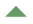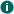9.2 Simple random samplingWhen we are interested in making inferences for a finite population and our sample begins to be a substantial proportion of that population then obviously our estimates will be more accurate and will tend to be perfect as we include more and more of the sample. In the case of simple random sampling there is a simple expression for how thedesign effects changes by having a finite population. If the sampling fraction is given by f then the design effect is reduced by a factor of (1-f). Thedesign factor is reduced by the square root of this. If we put some numbers into this expression we will see that we need to have quite a substantial f before the standard errors get much smaller. For example, for a sample 1:1000 the finite population correction (FPC) gives a reduction of only 0.005%. For 1:100 the reduction is 0.5% and one needs to go up to a sample of 1:10 before a 5% reduction in the standard error can be gained from the finite population correction. A sample of 1:2 will give us a FPC that reduces the standard error by about 30%. So it is clear from this that unless one's sampling fraction is 5% or more, it is quite unnecessary to include the FPC in the calculation of standard errors or design effects from surveys. These results apply only to simple random sampling in a single stage, not to two stage clustering sampling where the results are more complicated (see below).
 9.3 FPCs in stratified sampling When a survey is designed with stratification andFPC are appropriate they are applied within each stratum. When one or more strata have high sampling fractions this can result in a considerable reduction in the standard errors of the estimates. An example would be surveys of organisations where a there are a few very large organisations. The stratum for these organisations is often given a high sampling fraction, even 100% in some cases, because they would be such major contributors to population totals. See the section on disproportionate stratification for a discussion of this. If all of the units in a stratum are selected its results will be known exactly and so it will not contribute anything to the standard errors of means or totals. If the sampling fraction is high then their contribution to standard errors will be much reduced compared to simple random sampling.
 9.4 FPC in two-stage or multi-stahe sampling In two-stage sampling there will be different sampling fractions taken at each stage of the sampling. In this case the effect on the design effects and standard errors for surveys will be a complicated expression that involves the sampling fractions at each stage. In many cases the sampling fractions within individual clusters of a clustered sample will differ. For example if thePSUs are selected with probabilities proportional to size and then a fixed sample size is taken within each PSU, then the second stage sample fractions will be much larger in smaller PSUs. The way in which theFPC affect standard errors in this case will be a complicated expression and will depend on the extent to which the variables being analysed vary more within the PSUs or between PSUs. There are however one very common case where we don't need to worry about this. When the sampling fraction in the first stage (i.e. the sample of PSUs) is small enough to be ignored. If this is the case then the variation between the PSU means or totals will automatically incorporate any finite population correction that applies to the sub-sampling within the PSUs. Therefore if the first stage sampling fraction is small we can essentially ignore sub-sampling within the any later stages i.e. within clusters (PSUs). This is another advantage of the 'ultimate cluster' method of analysing clustered designs, see section 2.1 The only case we need to be concerned with is a two-stage design where we have sampled a substantial proportion of all the PSUs at the first stage and a substantial fraction of units at the second stage. The effect will be greatest where the variables being measured are highly variable between PSUs. In our experience this is uncommon, but a hypothetical example would be the following. A survey of primary school (P7) classes about what types of school meals they select with interest in this finite population here and now The primary sampling unit is schools, but in some schools there is more than one P7 class and to reduce work only a sample of classes is selected. The area being surveyed is divided into geographic strata and some strata have only a few schools, sometimes only a single school, so sampling fractions will be large. In these circumstances the finite population correction could make quite a big difference to the answers. To incorporate it fully you would need to use software that makes use of the full sampling design. SPSS, Stata version 9 and R allow you to do this. Overall, however, FPCs will usually be of minor importance to survey research and we have illustrated this in exemplar 4 and exemplar 5.